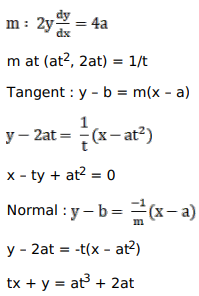# Find the equations of the tangent and the normal to the given curve at the indicated point for

Question:

Find the equations of the tangent and the normal to the given curve at the indicated point for

$\mathrm{y}^{2}=4 \mathrm{ax}$ at $\left(\mathrm{at}^{2}, 2 \mathrm{at}\right)$

Solution: